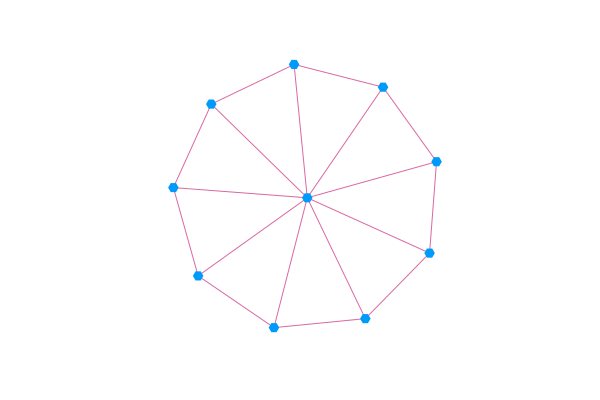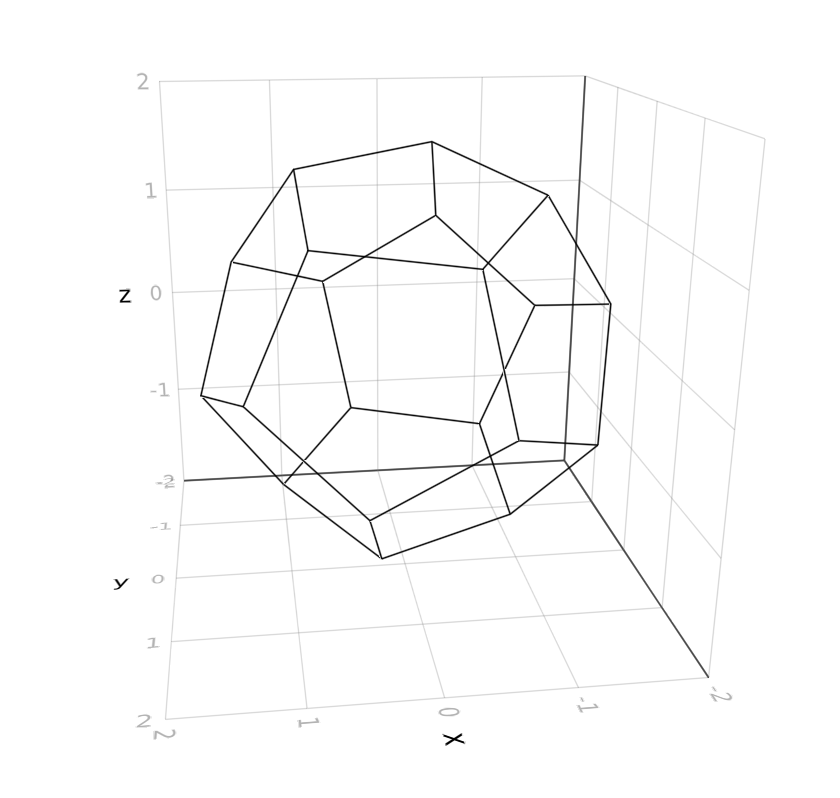Plotting Graphs

Graphs.jl integrates with several other Julia packages for plotting. Here are a few examples.

TikzGraphs.jl

Another nice graph visualization package. (TikzPictures.jl required to render/save):

julia> g = wheel_graph(10); t = plot(g)

julia> save(SVG("wheel10.svg"), t)

producing a graph like this:GraphPlot.jl

Another graph visualization package that is very simple to use. Compose.jl is required for most rendering functionality:

julia> using GraphPlot, Compose

julia> g = wheel_graph(10)

julia> draw(PNG("/tmp/wheel10.png", 16cm, 16cm), gplot(g))

SGtSNEpi.jl

SGtSNEpi.jl is a high-performance software for swift embedding of a large, sparse graph into a d-dimensional space (d = 1,2,3). The Makie plotting ecosystem is used for interactive plots.

using GLMakie, SGtSNEpi, SNAPDatasets

GLMakie.activate!()

y = sgtsnepi(g);
show_embedding(y;
A = adjacency_matrix(g),        # show edges on embedding
mrk_size = 1,                   # control node sizes
lwd_in = 0.01, lwd_out = 0.001, # control edge widths
edge_alpha = 0.03 )             # control edge transparency

The above code produces the following output:SGtSNEpi.jl enables 3D graph embedding as well. The 3D embedding of the weighted undirected graph ML_Graph/optdigits_10NN is shown below. It consists of 26,475 nodes and 53,381 edges. Nodes are colored according to labels provided with the dataset.GraphRecipes.jl

GraphRecipes.jl contains several recipes for plotting networks using the Plots.jl ecosystem. There are a lot of different recipes and styles to choose from, check out the examples page to see more of them.

using Plots, GraphRecipes
g = wheel_graph(10)
graphplot(g, curves=false)GraphMakie.jl

GraphMakie.jl is a graph plotting recipe for the Makie.jl ecosystem. It uses NetworkLayout.jl for the node placement and supports 2D and 3D plots. Check out the docs for more examples and tutorials.

using GLMakie, GraphMakie
using GraphMakie.NetworkLayout

g = smallgraph(:dodecahedral)
graphplot(g; layout=Stress(dim=3))